Google I/O is a wrap! Catch up on TensorFlow sessions

# Basics of machine learning with TensorFlow

This curriculum is for people who are:

• New to ML, but who have an intermediate programming background

This content is intended to guide developers new to ML through the beginning stages of their ML journey. You will see that many of the resources use TensorFlow, however, the knowledge is transferable to other machine learning frameworks.

## Step 1: Understand what ML is all about

TensorFlow 2.0 is designed to make building neural networks for machine learning easy, which is why TensorFlow 2.0 uses an API called Keras. The book Deep Learning with Python by Francois Chollet, creator of Keras, is a great place to get started. Read chapters 1-4 to understand the fundamentals of ML from a programmer's perspective. The second half of the book delves into areas like Computer Vision, Natural Language Processing, Generative Deep Learning, and more. Don't worry if these topics are too advanced right now as they will make more sense in due time.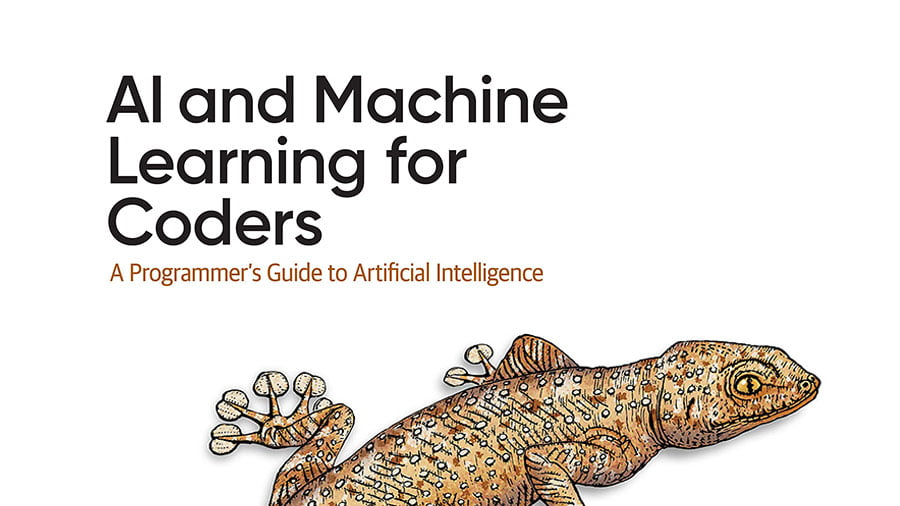AI and Machine Learning for Coders
by Laurence Moroney

This introductory book provides a code-first approach to learn how to implement the most common ML scenarios, such as computer vision, natural language processing (NLP), and sequence modeling for web, mobile, cloud, and embedded runtimes.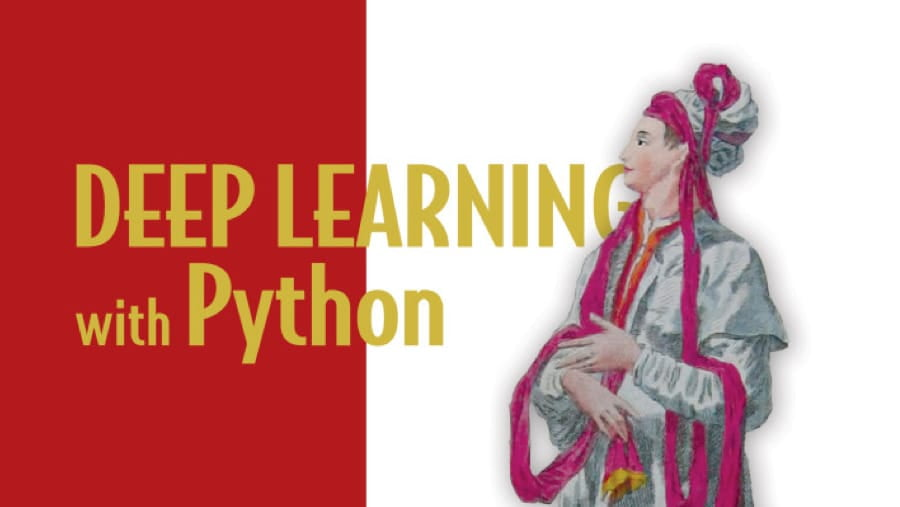Deep Learning with Python
by Francois Chollet

This book is a practical, hands-on introduction to Deep Learning with Keras.

##### ⬆ Or ⬇

Take an online course such as Coursera's Introduction to TensorFlow or Udacity's Intro to TensorFlow for Deep Learning, both of which cover the same fundamentals as Francois's book. You may also find these videos from 3blue1brown helpful, which give you quick explanations about how neural networks work on a mathematical level.

Completing this step will give you the foundations of how ML works, preparing you to go deeper.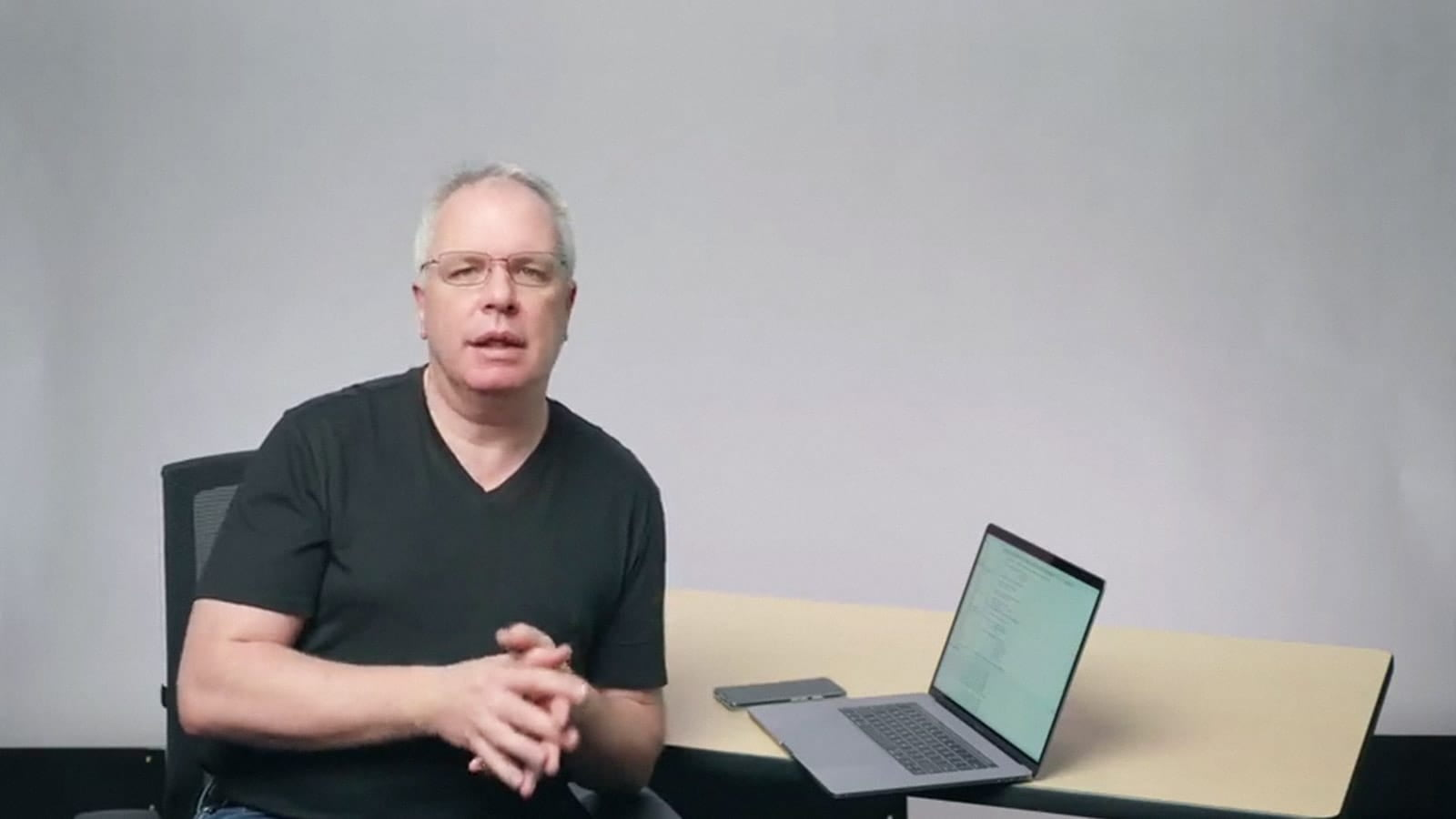Intro to TensorFlow for AI, ML, and Deep Learning

Developed in collaboration with the TensorFlow team, this course is part of the TensorFlow Developer Specialization and will teach you best practices for using TensorFlow.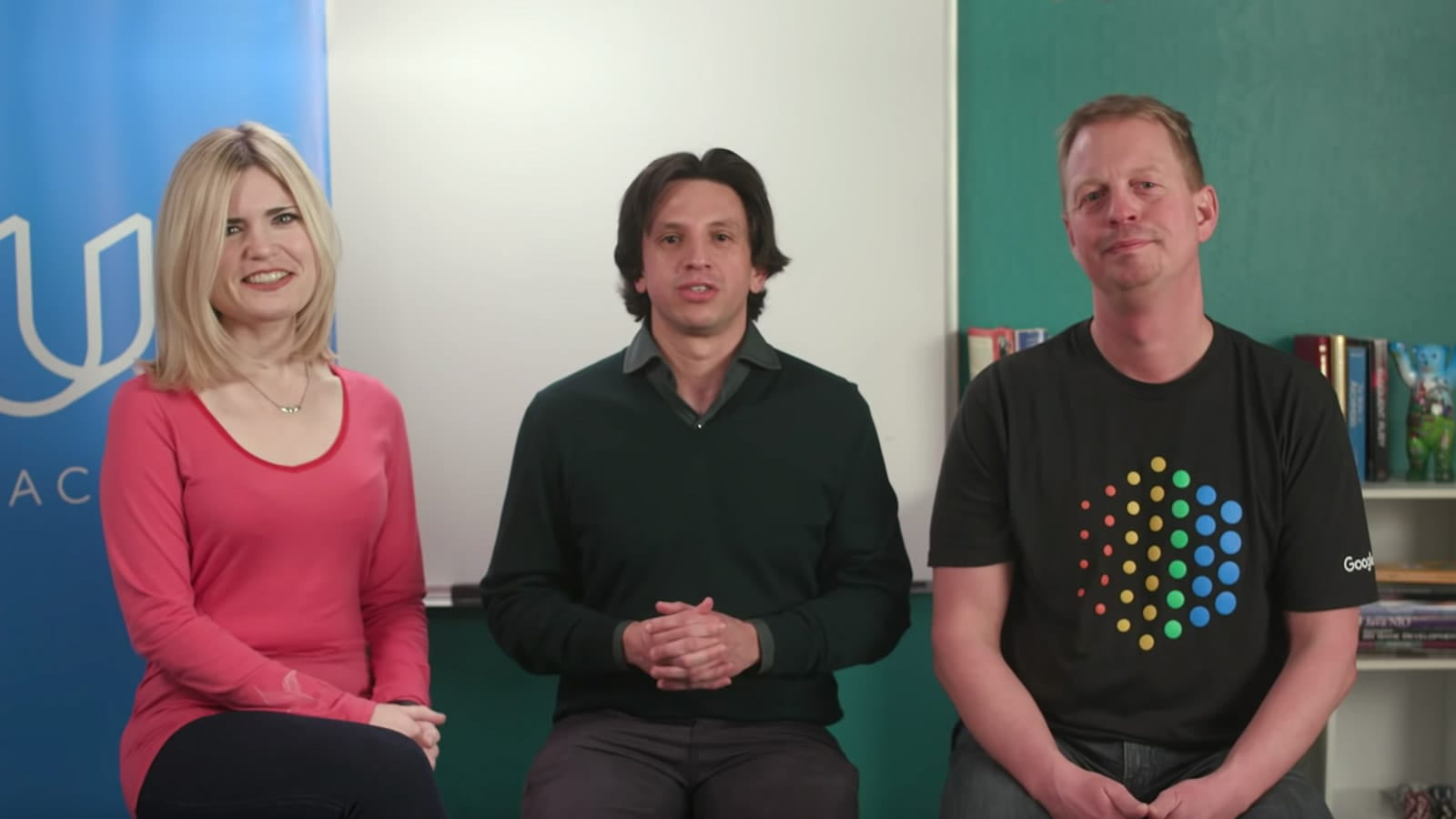Intro to TensorFlow for Deep Learning

In this online course developed by the TensorFlow team and Udacity, you'll learn how to build deep learning applications with TensorFlow.

## Step 2: Beyond the basics

Take the TensorFlow Developer Specialization, which takes you beyond the basics into introductory Computer Vision, NLP, and Sequence modelling.

Completing this step continues your introduction, and teaches you how to use TensorFlow to build basic models for a variety of scenarios, including image classification, understanding sentiment in text, generative algorithms, and more.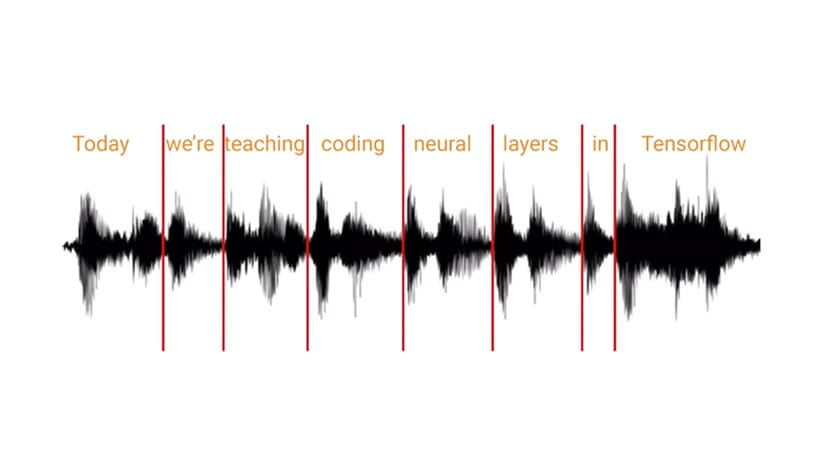TensorFlow Developer Specialization

In this four-course Specialization taught by a TensorFlow developer, you'll explore the tools and software developers use to build scalable AI-powered algorithms in TensorFlow.

## Step 3: Practice

Try some of our TensorFlow Core tutorials, which will allow you to practice the concepts you learned in steps 1 and 2. When you're done, try some of the more advanced exercises.

Completing this step will improve your understanding of the main concepts and scenarios you will encounter when building ML models.

## Step 4: Go deeper with TensorFlow

Now it's time to go back to Deep Learning with Python by Francois and finish chapters 5-9. You should also read the book Hands-on Machine Learning with Scikit-Learn, Keras, and TensorFlow, by Aurelien Geron. This book introduces ML and deep learning using TensorFlow 2.0.

Completing this step will round out your introductory knowledge of ML, including expanding the platform to meet your needs.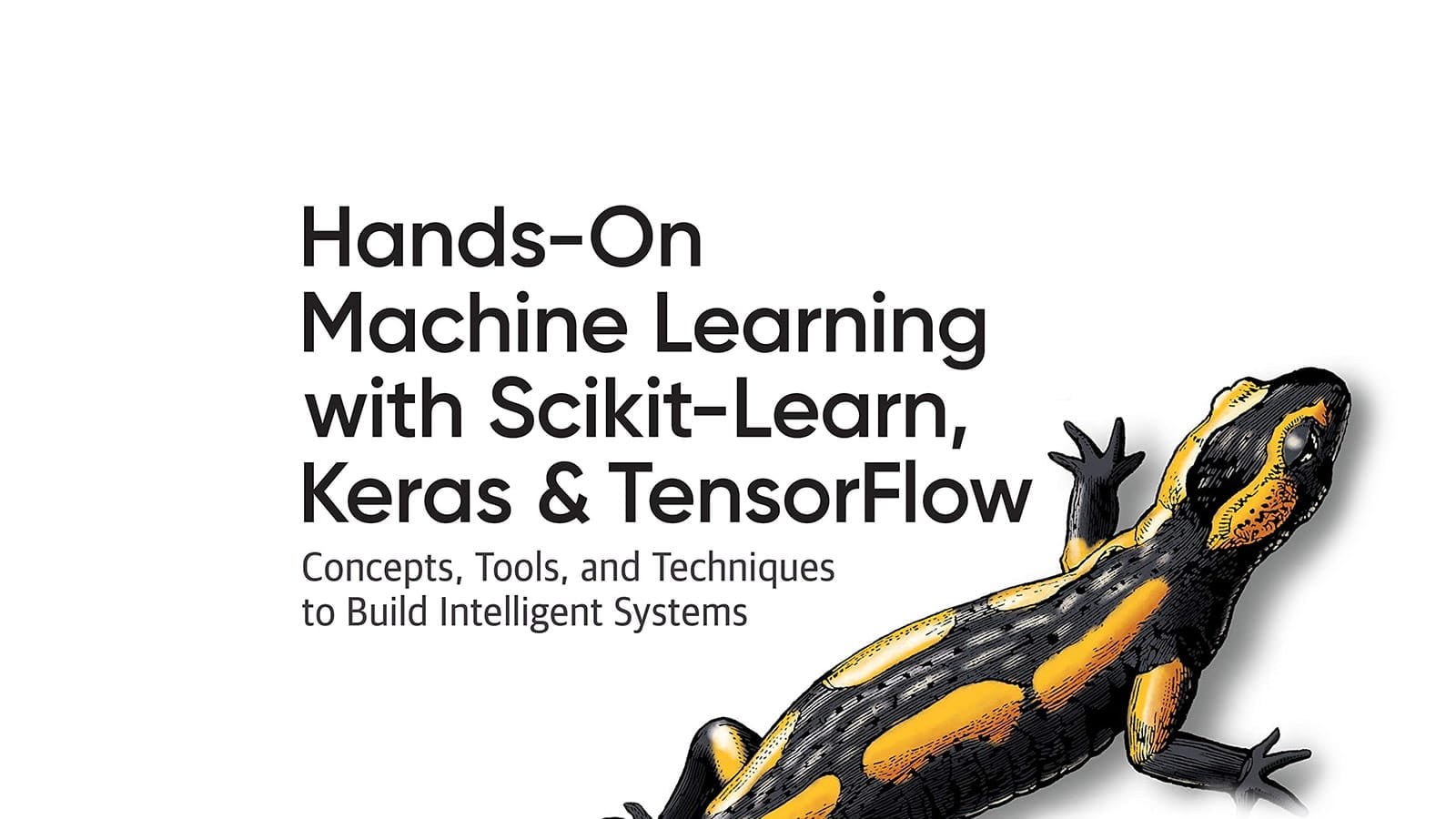Hands-on Machine Learning with Scikit-Learn, Keras, and TensorFlow
by Aurélien Géron

Using concrete examples, minimal theory, and two production-ready Python frameworks—Scikit-Learn and TensorFlow—this book helps you gain an intuitive understanding of the concepts and tools for building intelligent systems.

[]
[]